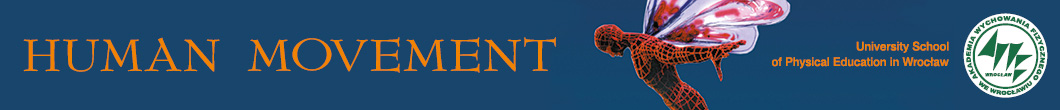ISSN: 1899-1955
Human Movement Special Issues
Current issue Archive Human Movement
5/2017

Share:
Share:
abstract:
Original paper

# The relative force in squat jump is the best laboratory predictor of sprint performance in adolescent soccer players

### Ladislav Dragula , Michal Lehnert , Rudolf Psotta , Zuzana Gonosová , Svatoslav Valenta , Petr Štastný

Online publish date: 2018/04/05
View full text Get citation

PlumX metrics:
Purpose
The aim was to determine the relationships between linear sprint performance and different outcomes relating to isokinetic strength, jump performance, and intermittent Wingate test performance in well-trained young (U19) soccer players.

Methods
The total of 18 elite male soccer players (age: 17.6 ± 0.8 years; body mass: 73.3 ± 5.9 kg, height: 180.8 ± 4.8 cm) performed a 20-m sprint test, countermovement jump, squat jump (SqJ), isokinetic measurement of knee flexors and extensors strength, and intermittent anaerobic test on a bicycle ergometer (IAnTB E10×6s with a 30-s rest interval). The strength and power outcomes of laboratory tests served as possible predictors of sprint performance in multiple regression analyses.

Results
The correlation analyses showed a significant relationship between all sprint lengths and relative maximum force measured by SqJ (r value: from –0.48 to –0.67) and between all sprint lengths and peak power (PP) measured by IAnTB E10×6s (r value: from –0.47 to –0.55). The average power measured by IAnTB E10×6s was associated with sprint lengths of 10, 15, and 20 m (r value: from –0.45 to –0.49). The SqJ predicted the 5-m sprint (R = 0.48; R2 = 0.18) and 10-m sprint (R = 0.55; R2 = 0.26). The PP together with the relative force (RF) predicted the 15-m (R = 0.65; R2 = 0.51) and 20-m (R = 0.77; R2 = 0.64) sprint performance.

Conclusions
The RF in SqJ can be used as a parameter relevant to linear 5-m, 10-m, 15-m, and 20-m sprint. Together with PP, it can be applied as a parameter relevant to linear 15-m and 20-m sprint.

keywords:

### acceleration, regression analyses, muscle strength, football

Termedia